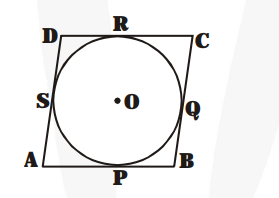# Prove that the parallelogram circumscribing a circle is a rhombus.`
Question:

Prove that the parallelogram circumscribing a circle is a rhombus.

Solution:

Let ABCD be a parallelogram such that its sides touch a circle with centre O.AP = AS [Tangents from an external point are equal]

BP = BQ

CR = CQ

DR = DS

$A P+B P+C R+D R=A S+D S+B Q+C Q$

$\mathrm{AB}+\mathrm{CD}=\mathrm{AD}+\mathrm{BC}$

2AB = 2BC

AB = BC

AB = BC = CD = DA

ABCD is a rhombus.

Hence proved